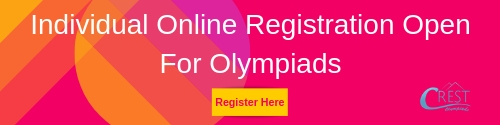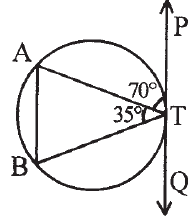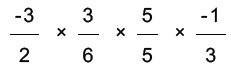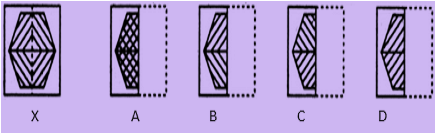ASSET - Math PDF Sample Papers for Class 10

Resources:

Class 10 sample paper & practice questions for ASSET Math are given below. Syllabus for level 1 is also mentioned for these exams. You can refer these sample paper & quiz for preparing for the exam.Sample Questions from Olympiad Success:

 Q.1 Q.2 Q.3 Q.4 Q.5 Q.6 Q.7 Q.8 Q.9 Q.10
 Q.1 The median of the triangle ABC meets at point G, which of these is not true? a) Area of triangle AGC = Area of triangle BGC b) Area of triangle AGC = Area of triangle AGB c) Area of triangle AGC = (1/3) Area of triangle ABC d) None of these
 Q.2 What will be the remainder if f(x) = x³ + 4x² – 3x + 1 is divided by (x – 2)? a) 18 b) 20 c) 19 d) 31
 Q.3 Two circles having same centres are called __________. a) incentric circles b) concentric circles c) centric circles d) Ellipse
 Q.4 Find out from the four alternatives as to how the pattern would appear when the transparent sheet is folded at the dotted line.a) A b) B c) C d) D
 Q.5 Find ∠BTQ?a) 30⁰ b) 45⁰ c) 75⁰ d) 90⁰
 Q.6 Find the following and simplify it to lowest term:a) 1/2 b) 1/6 c) 1/8 d) 1/4
 Q.7 T has child J. J has children M and N. M is married to C and has a child O. What is the relation between O and T? a) mother-in-law b) father-in-law c) grandparent d) great grandparent
 Q.8 Find the zeros of Polynomial in cx+d: a) -d/c b) d/c c) x/d d) c/d
 Q.9 Find out from the four alternatives as to how the pattern would appear when the transparent sheet is folded at the dotted line.a) B b) D c) C d) A
 Q.10 Which of these is false? a) The diagonal of the parallelogram divide the parallelogram into four equal parts b) Area of parallelogram is base multiplied by the height c) If EFGH are the respective midpoints of the sides of the parallelogram ABCD, Area of EFGH is half the area of the parallelogram d) None of theseSample PDF of ASSET - ASSET Math PDF Sample Papers (MATH) for Class 10: